### Transformations of the parabola

#### Translations

We can translate the parabola vertically to produce a new parabola that is similar to the basic parabola. The function $$y=x^2+b$$ has a graph which simply looks like the standard parabola with the vertex shifted $$b$$ units along the $$y$$-axis. Thus the vertex is located at $$(0,b)$$. If $$b$$ is positive, then the parabola moves upwards and, if $$b$$ is negative, it moves downwards.

Similarly, we can translate the parabola horizontally. The function $$y=(x-a)^2$$ has a graph which looks like the standard parabola with the vertex shifted $$a$$ units along the $$x$$-axis. The vertex is then located at $$(a,0)$$. Notice that, if $$a$$ is positive, we shift to the right and, if $$a$$ is negative, we shift to the left.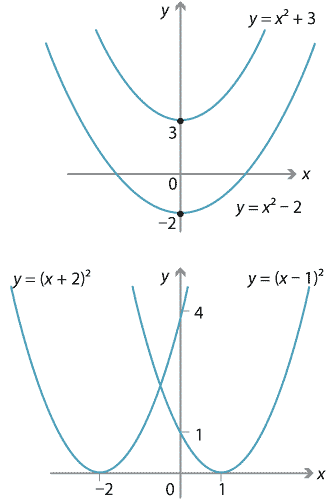Detailed description of diagram

These two transformations can be combined to produce a parabola which is congruent to the basic parabola, but with vertex at $$(a,b)$$.

For example, the parabola $$y=(x-3)^2+4$$ has its vertex at $$(3,4)$$ and its axis of symmetry has the equation $$x=3$$.

In the module Algebra review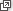, we revised the very important technique of completing the square. This method can now be applied to quadratics of the form $$y=x^2+qx+r$$, which are congruent to the basic parabola, in order to find their vertex and sketch them quickly.

#### Example

Find the vertex of $$y=x^2-4x+8$$ and sketch its graph.

#### Solution

Completing the square, we have

\begin{align*} y &= x^2-4x+8\\ &= (x-2)^2+4. \end{align*}

Hence the vertex is $$(2,4)$$ and the axis of symmetry has equation $$x=2$$.

The graph is shown below.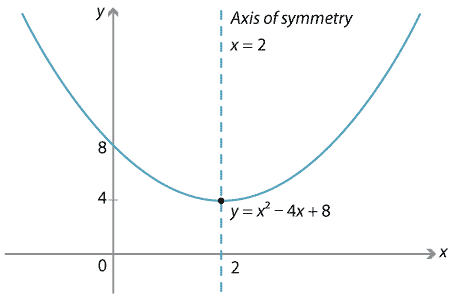Detailed description of diagram

We can, of course, find the vertex of a parabola using calculus, since the derivative will be zero at the $$x$$-coordinate of the vertex. The $$y$$-coordinate must still be found, and so completing the square is generally much quicker.

#### Reflections

Parabolas can also be reflected in the $$x$$-axis. Thus the parabola $$y=-x^2$$ is a reflection of the basic parabola in the $$x$$-axis.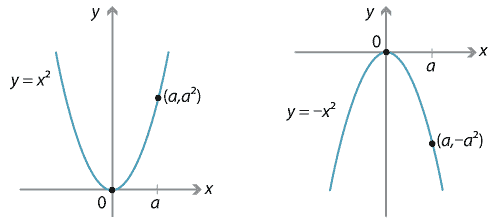Detailed description of diagrams

#### Example

Find the vertex of $$y=-x^2+6x-8$$ and sketch its graph.

#### Solution

Completing the square, we have

\begin{align*} y &= -\big(x^2-6x+8\big)\\ &= -\big((x-3)^2-1\big) = 1-(x-3)^2. \end{align*}

Hence the vertex is $$(3,1)$$ and the parabola is 'upside down'. The equation of the axis of symmetry is $$x=3$$. The graph is shown below.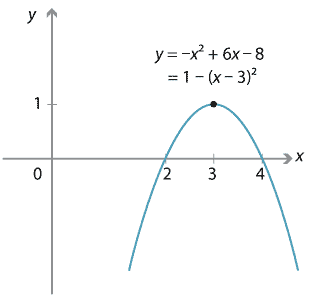Detailed description of diagram

##### Exercise 1

Find the vertex of each parabola and sketch it.

1. $$y=x^2+x+1$$
2. $$y= -x^2-6x-13$$

#### Stretching

Not all parabolas are congruent to the basic parabola. For example, the arms of the parabola $$y=3x^2$$ are steeper than those of the basic parabola. The $$y$$-value of each point on this parabola is three times the $$y$$-value of the point on the basic parabola with the same $$x$$-value, as you can see in the following diagram. Hence the graph has been stretched in the $$y$$-direction by a factor of 3.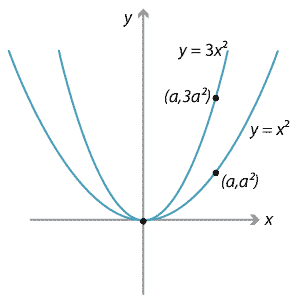Detailed description of diagram

In fact, there is a similarity transformation that takes the graph of $$y=x^2$$ to the graph of $$y=3x^2$$. (Map the point $$(x,y)$$ to the point $$(\dfrac{1}{3}x, \dfrac{1}{3}y)$$.) Thus, the parabola $$y=3x^2$$ is similar to the basic parabola.

In general, the parabola $$y=ax^2$$ is obtained from the basic parabola $$y=x^2$$ by stretching it in the $$y$$-direction, away from the $$x$$-axis, by a factor of $$a$$.

##### Exercise 2

Sketch the graphs of $$y=\dfrac{1}{2}x^2$$ and $$y=x^2$$ on the same diagram and describe the relationship between them.

This transformation of stretching can now be combined with the other transformations discussed above. Once again, the basic algebraic technique is completing the square.

#### Example

Find the vertex of the parabola $$y=2x^2+4x+9$$ and sketch its graph.

#### Solution

By completing the square, we obtain

\begin{align*} y &= 2x^2+4x+9 \\ &= 2\Big(x^2 + 2x + \dfrac{9}{2}\Big)\\ &= 2\Big((x+1)^2 + \dfrac{7}{2}\Big)\\ &= 2(x+1)^2+7. \end{align*}

Hence the vertex is at $$(-1,7)$$.

The basic parabola is stretched in the $$y$$-direction by a factor of 2 (and hence made steeper) and translated.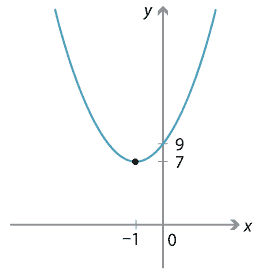Detailed description of diagram

#### Rotations

The basic parabola can also be rotated. For example, we can rotate the basic parabola clockwise about the origin through $$45^\circ$$ or through $$90^\circ$$, as shown in the following two diagrams.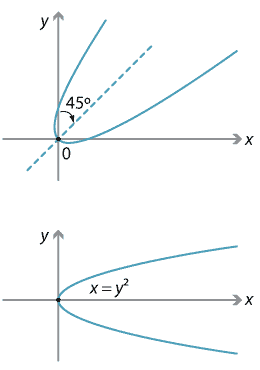Detailed description of diagrams

Algebraically, the equation of the first parabola is complicated and generally not studied in secondary school mathematics.

The second parabola can be obtained from $$y=x^2$$ by interchanging $$y$$ and $$x$$. It can also be thought of as a reflection of the basic parabola in the line $$y=x$$. Its equation is $$x=y^2$$ and it is an example of a relation, rather than a function (however, it can be thought of as a function of $$y$$).

#### Summary

All parabolas can be obtained from the basic parabola by a combination of:

• translation
• reflection
• stretching
• rotation.

Thus, all parabolas are similar.

This is an important point, since in the module Polynomials, it is seen that the graphs of higher degree equations (such as cubics and quartics) are not, in general, obtainable from the basic forms of these graphs by simple transformations. The parabola, and also the straight line, are special in this regard.

For the remainder of the Content section of this module, we will restrict our attention to parabolas whose axis is parallel to the $$y$$-axis. It will be to these types that we refer when we use the term 'parabola'.

Next page - Content - Intercepts